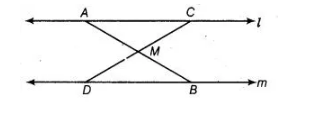# In given figure $l | m$ and $M$ is the mid-point of a`
Question:

In given figure $l \| m$ and $M$ is the mid-point of a line segment $A B$. Show that $M$ is also the mid-point of any line segment $C D$, having its end points on $I$ and $m$, respectively.Solution:

Given In the figure, $l \| m$ and $M$ is the mid-point of a line segment $A B$ i.e., $A M=B M$.

To show $M C=M D .$

Proof $\because$      $l \| m$    [given]

$\angle B A C=\angle A B D$     [alternate interior angles]

$\angle A M C=\angle B M D$ [vertically opposite angles]

In $\triangle A M C$ and $\triangle B M D$, $\angle B A C=\angle A B D$ [proved above]

$A M=B M$ [given]

and $\angle A M C=\angle B M D$ [proved above]

$\therefore$ $\triangle A M C \cong \triangle B M D$ [by ASA congruence rule)

$\Rightarrow$ $M C=M D$ [by CPCT]